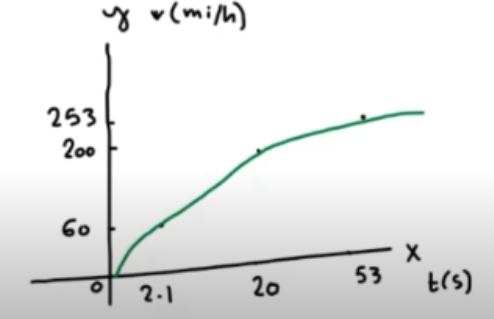Need Help?

Subscribe to Physics 1

###### \${selected_topic_name}
• Notes
• Comments & Questions

$\begin{array}{l}{\text { A car travels in the +x-direction on a straight and level }} \\ {\text { road. For the first } 4.00 \text { s of its motion, the average velocity of the }} \\ {\text { car is } \mathrm{Vav}-\mathrm{x}=6.25 \mathrm{m} / \mathrm{s} \text { . How far does the car travel in } 4.00 \mathrm{s} ?}\end{array}$

$V{a v-x}=\frac{\Delta x}{\Delta t}$

$\longrightarrow \Delta X=V{a v-x} \quad \Delta t$

$=6.25 *(4)=25 m$

$\begin{array}{l}{\text { Starting from the front door of your ranch house, you walk } 60.0 \mathrm{m} \text { due east to your windmill, and then you turn }} \\ {\text { around and slowly walk } 40.0 \mathrm{m} \text { west to a bench where you sit and watch the sunrise. It takes you } 28.0 \text { sto walk }} \\ {\text { from your house to the windmill and then } 36.0 \text { s to walk from the windmill to the bench. For the entire trip from }} \\ {\text { your front door to the bench, what are (a) your average velocity and (b) your average speed? }}\end{array}$

$V{a v-x}=\frac{\Delta x}{\Delta t} \quad, \quad \text { avg speed }=\frac{\text { Total Disit }}{\text { Total time }}$

a) $le t+x \text { be east } \rightarrow \Delta x=60-40=20 \mathrm{m}\quad, \ \Delta t=28+36=64 \mathrm{s}$

$\rightarrow Vav-{x}=\frac{\Delta x}{\Delta t}=\frac{20}{64}=0.312 \mathrm{m} / \mathrm{s}$

b) $\text { avg speed }=\frac{60+40}{64}=1.56 \mathrm{m} / \mathrm{s}$

$\begin{array}{l}{\text { The rasteri (and Most Expensive) carl The table stest data for the Bugattiverron, the tates tar made. The carls moving in astraight line }} \\ {\text { (the } x \text { -axis). }}\end{array}$

$\begin{array}{llll}{\text { Time (s) }} & {0} & {\text { 2.1 }} & {20.0} & {53} \\ {\text { Speed }(\mathrm{mi}>\mathrm{h})} & {0} & {60} & {200} & {253} \\ {(\mathrm{m} / \mathrm{s})} & {0} & {26.82} & {89.4} & {\mathrm{ll} 3.1}\end{array}$

$\text { (a) Make a Vx-t graph of this car's velocity (in } \mathrm{mi} / \mathrm{h}) \text { as a function of time. }$

$\text { Is its acceleration constant? (b) Calculate the car's average acceleration }$

$(\text { in } \mathrm{m} / \mathrm{s} 2 \text { ) between }(\mathrm{i}) 0 \text { and } 2.1 \mathrm{s} ;(\mathrm{i} \mathrm{i}) \text { 2.1 } \mathrm{s} \text { and } 20.0 \mathrm{s} ;(\mathrm{iii}) 20.0 \mathrm{s} \text { and } 53 \mathrm{s}$

$\text { Are these results consistent with your graph in part (a)? (Before you }$

$\begin{array}{l}{\text { decide to buy this car, it might be helptur that only } 300 \text { will be built, it runs out of gas in } 12 \text { minutes at top speed, and it costs } \ 1.25} \\ {\text { millionly }}\end{array}$

$1mi/h=0.447 \mathrm{m/s}$(i) $a_{a v-x}=\frac{26.82-0}{2.1}=12.8 \mathrm{m/s}^{2}$

(ii) $a_{av-x}=\frac{89.4-26.82}{20-2.1}=3.5 \mathrm{m} / \mathrm{s}^{2}$

(iii)$a_ \text { av-x }=\frac{113.1-89.4}{53-20}=0.718 \mathrm{m/s}^{2}$

$a_{a v-x}=\frac{113.1-0}{53}=2.13 \mathrm{m} / \mathrm{s}^{2}$

No comments yet